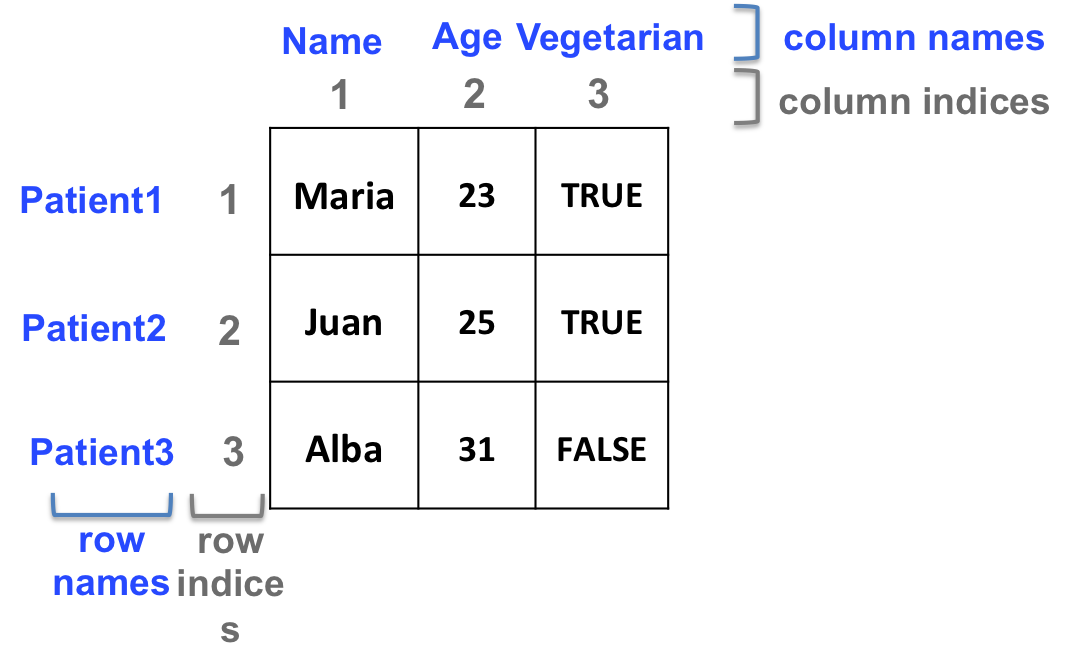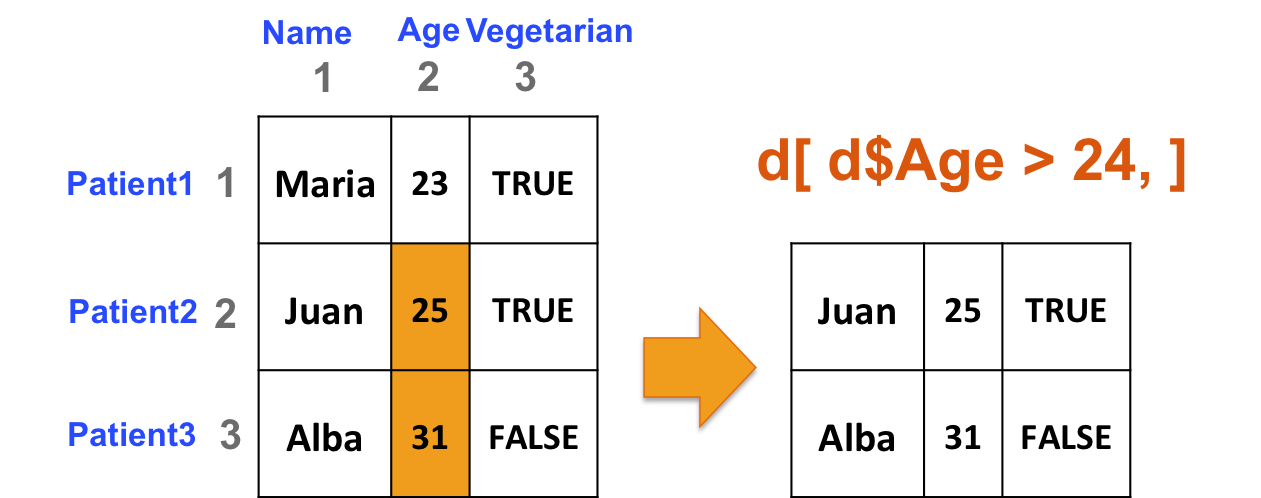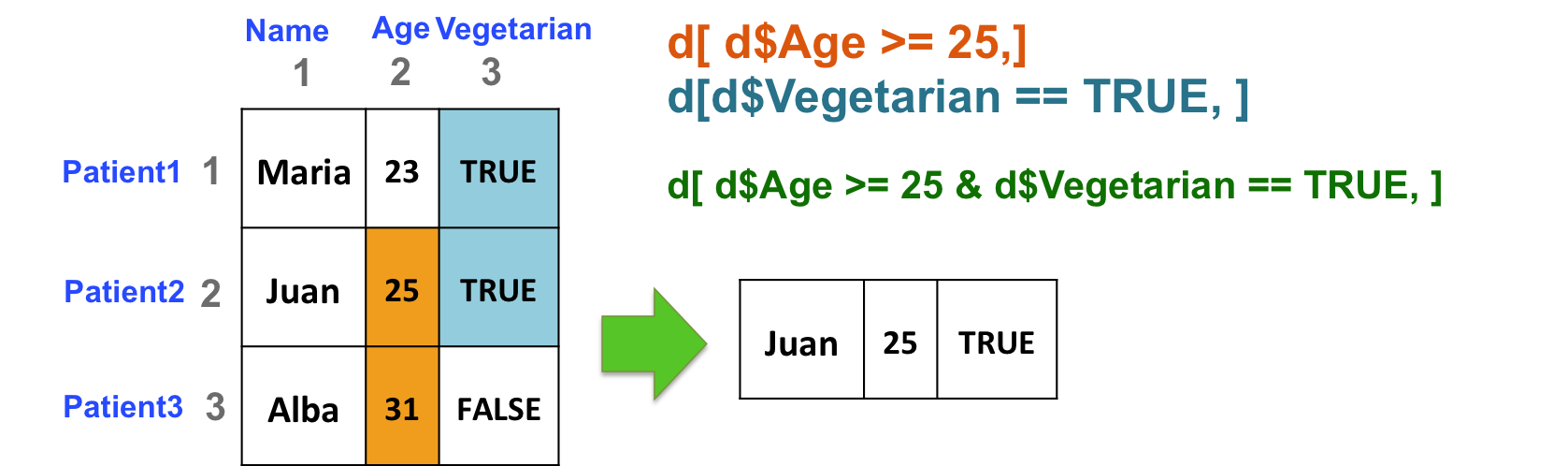## 9.7 Two-dimensional structures manipulation

### 9.7.1 Dimensions

• Get the number of rows and the number of columns:
``````# Create a data frame
d <- data.frame(c("Maria", "Juan", "Alba"),
c(23, 25, 31),
c(TRUE, TRUE, FALSE),
stringsAsFactors = FALSE)
# number of rows
nrow(d)
# number of columns
ncol(d)``````
• Check the dimensions of the object: both number of rows and number of columns:
``````# first element: number of rows
# second element: number of columns
dim(d)``````
• Dimension names

Column and/or row names can be added to matrices and data frames

``````colnames(d) <- c("Name", "Age", "Vegetarian")
rownames(d) <- c("Patient1", "Patient2", "Patient3")``````

Column and/or row names can be used to retrieve elements or sets of elements from a 2-dimensional object:``````d[,"Name"]
# same as:
d[,1]

d["Patient3", "Age"]
# same as:
d[3,2]

# for data frames only, the \$ sign can be used to retrieve columns:
# d\$Name is d[,1] is d[, "Name"]``````
• Include names as you create objects:
• Matrix:
``````m <- matrix(1:4, ncol=2,
dimnames=list(c("row1", "row2"), c("col1", "col2")))``````
``+ Data frame:``
``````df <- data.frame(col1=1:2, col2=1:2,
row.names=c("row1", "row2"))``````

### 9.7.2 Manipulation

Same principle as vectors… but in 2 dimensions!

#### 9.7.2.1 Examples

• Select rows of b if at least one element in column 2 is greater than 24 (i.e. select patients if they are older than 24):
``````# build data frame d
d <- data.frame(Name=c("Maria", "Juan", "Alba"),
Age=c(23, 25, 31),
Vegetarian=c(TRUE, TRUE, FALSE),
stringsAsFactors = FALSE)
rownames(d) <- c("Patient1", "Patient2", "Patient3")
# The following commands all output the same result:
d[d[,2] > 24, ]
d[d[,"Age"] > 24, ]
d[d\$Age > 24, ]``````• Select patients (rows) based on 2 criteria: age of the patient (column 2) should be great than or equal to 25, and the patient should be vegetarian (column 3):
``d[ d\$Age >= 25 & d\$Vegetarian == TRUE, ]``• Select the columns of b if at least one element in the 3rd row is less than or equal to 4:
``````# create b
b <- matrix(c(1, 0, 34, 44, 12, 4),
nrow=3,
ncol=2)
# third row of b:
b[3, ]
# element(s) in the third row of b that is (are) less than or equal to 4
b[3, ] <= 4
# retrieve the corresponding sub-matrix
b[ ,b[3, ] <= 4]``````

#### 9.7.2.2 More useful commands

• Add a row or a column with rbind and cbind, respectively
``````# add a column
cbind(d, 1:3)
rbind(d, 4:6)``````

Add a patient to our data frame d:

``d <- rbind(d, c("Jordi", 33, FALSE))``
• Process the sum of all rows or all columns with rowSums and colSums, respectively.
``````# create a matrix
b <- matrix(1:20, ncol=4)
# process sum of rows and sum of cols
rowSums(b)
colSums(b)``````
• The apply function

Powerful tool to apply a command to all rows or all columns of a data frame or a matrix.
For example, instead of calculating the sum of each row, you might be interested in calculating the median ? But rowMedians doesn’t exist !

apply takes 3 arguments:

• first argument X: 2-dimensional object
• second argument MARGIN: apply by row or by column?
• 1: by row
• 2: by column
• third argument FUN: function to apply to either rows or columns
``````# median value of each row of b
apply(X=b, MARGIN=1, FUN=median)
# median value of each column of b
apply(X=b, MARGIN=2, FUN=median)``````